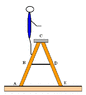# Student on Ladder - Equilibrium Problem

In summary, the student is standing on a ladder with weight W and tension T. The normal forces on the ladder legs are Nl and Nr. The student is standing at a spot 2.025 m along the leg and her weight is 466 N.f

This problem has been posted here a couple of times, but I can't finish it off, due to some combination of my failure to sum the torques and working out the geometry.

## Homework Statement

A student is standing on a ladder as shown in the figure to the right. Each leg of the ladder is 2.7 m long and is hidged at point C. The tie-rod (BD) attached halfway up and is 0.72 m long. The student is standing at a spot 2.025 m along the leg and her weight is 466 N. (You may ignore the weight of the ladder and any minor friction between the floor and the legs.)

## Homework Equations

Sum of Forces = 0
Sum of Torques = 0
W = weight of student
T = Tension
Nl = Normal force at point A on left leg
Nr = Normal force at point E on right leg
Cr, Cl = The forces exerted on the ladder legs by each other respectively at point C.

## The Attempt at a Solution

I started by calculating θ (the angle at a) as sin θ = .36/1.35. Is this the proper way to do this? I'm not sure if I can say that the length of the ladder leg is 1.35 halfway up the vertical height. I found θ=74.534

I then summed torques about point C.

(T(2.7/2)sin θ) - (W(2.025)cos θ) - (Nl(2.7)cos θ) = 0
Nl = 1.8071T - 349.5

I then plugged this equation into
T-Clx = 0 and Cly + Nl - W = 0
in an attempt to solve for T, but am unable to get the correct answer.

I'm not sure if I've set up the torque equation properly or if I've performed the trigonometry correctly to find the angles. I'd greatly appreciate any guidance here.

#### Attachments

•showme.gif
2.2 KB · Views: 599

What is the question?

ehild

Oops, I have to solve for the tension in the center rod and the Normal forces at points a and E. If I could just get one of those the others would come easily.

I started by calculating θ (the angle at a) as sin θ = .36/1.35. Is this the proper way to do this? I'm not sure if I can say that the length of the ladder leg is 1.35 halfway up the vertical height. I found θ=74.534

If sin θ = .36/1.35θ than θ is half the angle at C the legs enclose, or the angle one leg makes with the vertical. But it is not 74.534° -you hit inverse-cosine instead of sine, I am afraid. So decide, which angle do you use.

ehild

Sorry, I meant cos θ = .36/1.35 = 74.534°. I am pretty sure this is the angle I want for my torque equation.

Is the student standing at 2.025 m distance from C or from A? If he stands above the tie-rod, the torque is not correct.

What did you denote by x?

ehild

Clx is the force applied to the left leg at C in the x direction.

Thanks for pointing out the distance mistake, I'll correct that and see how it goes.

You have the other leg, too...

ehild

I'm afraid I don't understand what you mean. Do I have to include the torques acting on the right leg in my equation? I thought that I would be able to set up an equation for the torques acting on the left leg and proceed from there.

This is the equation I'm currently working with to find the torques acting on the left leg, is it correct?

(T(2.7/2)sin θ) - (W(2.7-2.025)cos θ) - (Nl(2.7)cos θ) = 0

Do I need to set up another torque equation for the right leg and equate the two?

If so, I set up the torques for the right leg as follows:
(Nl(2.7)cos θ) - (T(2.7/2)sin θ) = 0

I solved each equation for Nl and Nr, then plugged these values into Nr + Nl = W to solve for T.
1.8071T + (1.8071T - 116.5) = 466
Congrats!